# Analysis Of Correlation Between Leo Token and Ethereum

I considered finding the relationship between that of bitcoin and that of leo token in my previous post and the result indicated a medium positive relationship between the two tokens.

Meaning that as bitcoin climbs up in price, it is more likely that the price of leo token will climb and vice versa.

This article will analyze the relationship between the price of leo token and that of ethereum. I suggest that analyst in this community also give their suggestion concerning the analysis presented below and what they think about it.

In the course of this article, I intend to cover up the following areas of concern, brief introduction to analysis of correlation, hypothesis and theories of correlation test, data presentation of prices of leo token and ethereum, analysis and presentation of result of relationship.

Introduction to analysis of correlation
Analysis of correlation will find the relationship between more than one variable and how they relate in a process.
The variables considered in this case are the prices of leo token and that of ethereum using the data from coingecko for the past 14 days.

Hypothesis and theories of correlation

Hypothesis
Hypothesis is a statement representing a general fact concerning a particular interest.
There are two kinds of hypothesis namely; the null hypothesis and the alternate hypothesis.

The null hypothesis for correlation test states that there is no relationship between the variables in consideration (price of leo token to that of ethereum).

The alternate hypothesis states that there is a relationship between price of leo token and that of etheruem.

Whether there is a relationship or not, we shall find out in our result.

Secondly, the correlation coefficient is determined by considering the following fact.

Negative correlation
Variables are negatively correlated when an increase in one will lead to a simultaneous decrease in the other. Such correlation is said to be a negative one and tends towards -1 on the number scale system.

Positive correlation
A positive correlation indicates that the higher one variable, the higher the other variable. This entails the coefficient of correlation is positive as well and tends towards a positive one on the number scale system.

Zero correlation
Zero correlation indicates that there is no relationship at all between the variables in consideration and the correlation coefficient on a number line tends towards zero from the direction of either positive or negative ones.

The coefficient of correlation resides on a line of -1 to 0 to +1 as seen below.
-1____________________0__________________+1

The data below shows the price of leo token and ethereum from May 2nd to May 16th, 2022. It was entered using the variable and data view mode of SPSS.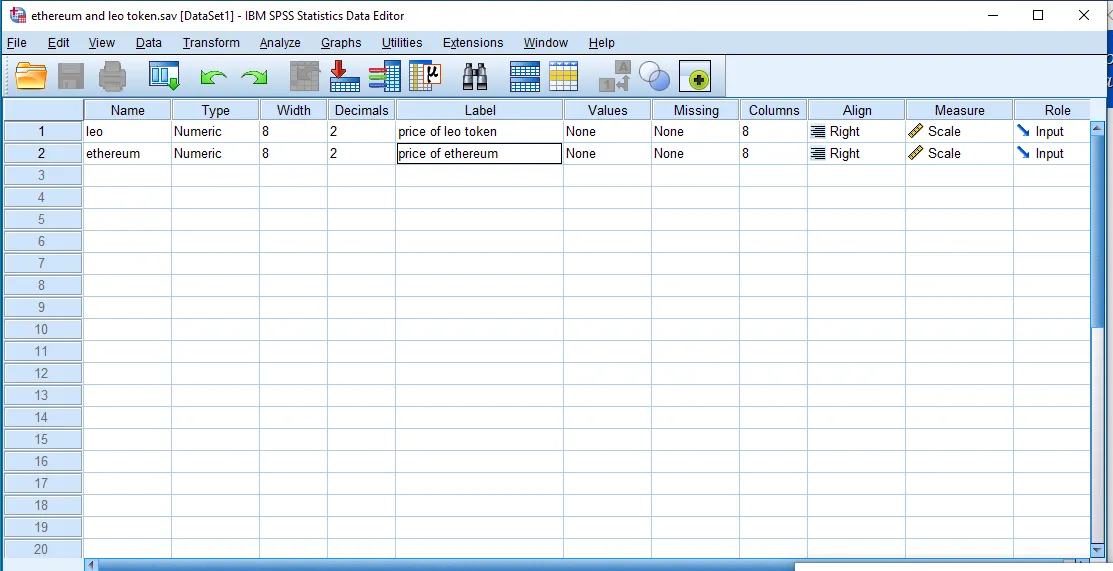Taken With Desktop

We shall be making use of 61 sample prices from coin gecko.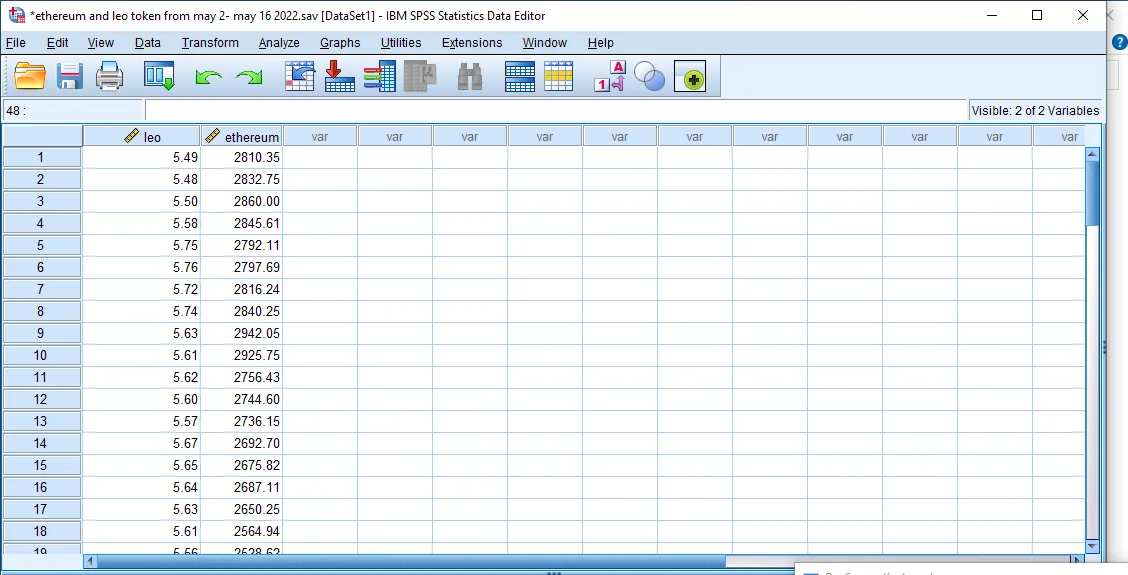Taken With Desktop

We shall consider first the nature or direction of the relationship between the prices if it tends towards -1 or +1 or even 0.

In order to view this, we shall use the scatter plot diagram which can be seen below.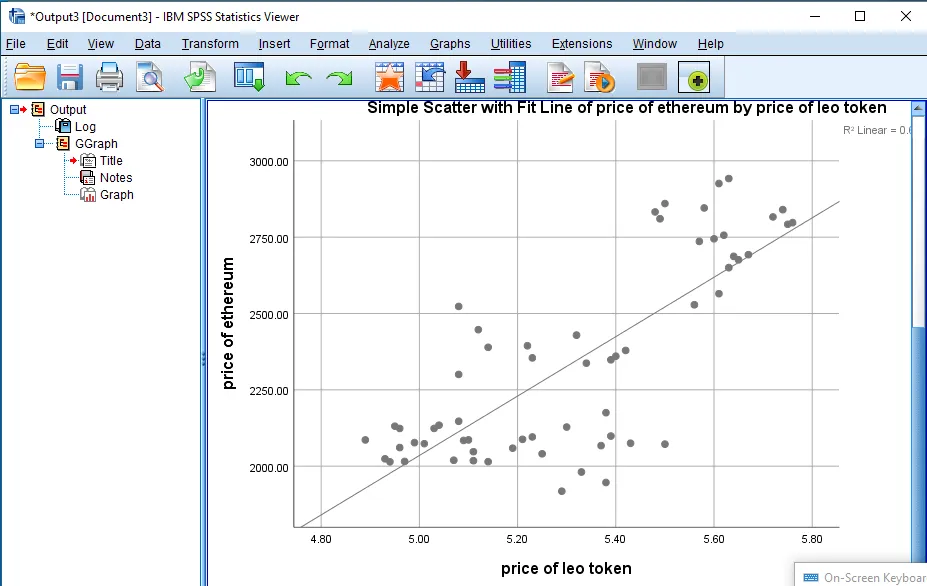Taken With Desktop

From the scattered diagram we can see a maximum positive relationship between the price of ethereum and leo token.

We can proceed to the actual relationship which can be seen below.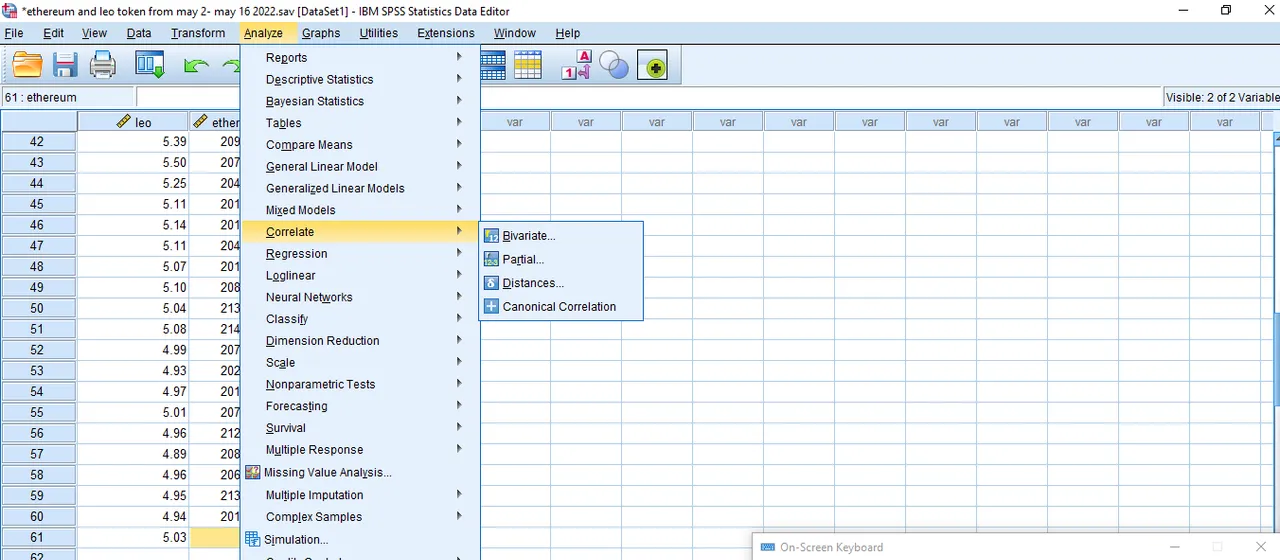Taken With Desktop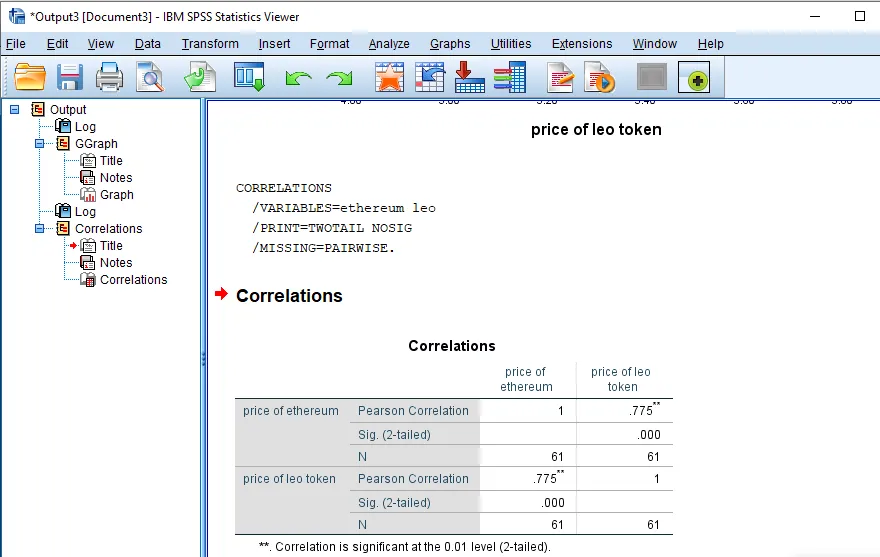Taken With Desktop

It is visible that our coefficient of correlation tends towards the positive axis of +1. This indicates a very good relationship between the price of ethereum and that of leo token. In a nutshell, when the price of ethereum goes up the price of leo token will also go up.

Considering a significant level of 0.00 which is lesser that 0.05, we can reject the null hypothesis which states there is no relationship between the prices of both tokens and there is a significant difference in the stated hypothesis

Conclusion
This article has been able to analyze the data of the prices of Leo token with that of ethereum which indicates a positive relationship as well.

H2
H3
H4
3 columns
2 columns
1 column
1 Comment
Ecency